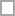# Algebraic Reasoning Quizzes & Trivia

Curious and eager to learn new trivia about life, the universe, and everything? If yes, what better way to take some awesome algebraic reasoning quizzes online? Test yourself and share these algebraic reasoning quizzes to find out who is the quiz champ!

Enhance your knowledge about a topic or learn something completely new by answering ultimate algebraic reasoning quiz questions.

Each and every algebraic reasoning quiz that we have is made up of well-researched and interesting quiz questions. With detailed instant feedback for quiz answers, you can easily learn something new about algebraic reasoning with every question you attempt.

Questions: 10  |  Attempts: 244   |  Last updated: Oct 7, 2013
• Sample Question
Which of the following statements best describes the data in the table below?Questions: 5  |  Attempts: 138   |  Last updated: Oct 9, 2013
• Sample Question
Let n represent the position of a number in the following arithmetic sequence.  4, 10, 16, 22 ....To allow students to show they understood the presentation.

Questions: 5  |  Attempts: 18   |  Last updated: Dec 31, 2012
• Sample QuestionAlgebraic reasoning is a process of generalizing mathematical operations. What do you know about it? Is your knowledge solid? Alright geeksters, take this quiz to know where you stand.

Questions: 10  |  Attempts: 10   |  Last updated: Nov 28, 2017
• Sample Question
Algebraic reasoning involves operating onRelated

## Algebraic Reasoning Questions and Answers

• Which of the following statements best describes the data in the table below?Algebraic reasoning question from

• Which of the following statements best describes the data in the table below?Algebraic reasoning question from

• Which of the following statements best describes the data in the table below?Algebraic reasoning question from

• Let n represent the position of a number in the following arithmetic sequence.  4, 10, 16, 22 ....Algebraic reasoning question from

• A sequence of numbers was formed using the rule  -3n+4, where n represents the number's position in a sequence. Which sequence fits this rule?Algebraic reasoning question from

• Carla's Day Care Center charges \$4 per hour ot babysit plus a \$10 fee for meals.  She uses the equation below to calculate c, her total charge based on h, the number of hours she babysits. c = 10 + 4h How much will Carla charge if she babysits for 3 hours 30 minutes?Algebraic reasoning question fromAlgebraic reasoning question from

• How many steps are there for implementing algebraic reasoning into the elementary classroom?Algebraic reasoning question from

• What did the example used in the article include?Algebraic reasoning question from

• Algebraic reasoning involves operating onAlgebraic reasoning question from

• Algebraic reasoning employs the discourse ofAlgebraic reasoning question from

• Which of the following can be used to represent generalisations in algebra?Algebraic reasoning question from